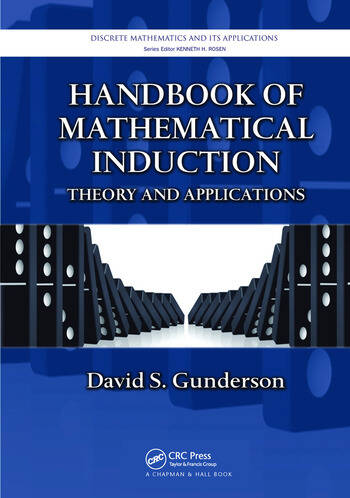# Handbook of Mathematical Induction: Theory and Applications

## 1st Edition

David S. Gunderson

Chapman and Hall/CRC
Published November 16, 2016
Reference - 921 Pages - 38 B/W Illustrations
ISBN 9781138199019 - CAT# K31433
Series: Discrete Mathematics and Its Applications

For Instructors Request Inspection Copy

was \$64.95

USD\$51.96

SAVE ~\$12.99

FREE Standard Shipping!

## Preview

### Summary

Handbook of Mathematical Induction: Theory and Applications shows how to find and write proofs via mathematical induction. This comprehensive book covers the theory, the structure of the written proof, all standard exercises, and hundreds of application examples from nearly every area of mathematics.

In the first part of the book, the author discusses different inductive techniques, including well-ordered sets, basic mathematical induction, strong induction, double induction, infinite descent, downward induction, and several variants. He then introduces ordinals and cardinals, transfinite induction, the axiom of choice, Zorn’s lemma, empirical induction, and fallacies and induction. He also explains how to write inductive proofs.

The next part contains more than 750 exercises that highlight the levels of difficulty of an inductive proof, the variety of inductive techniques available, and the scope of results provable by mathematical induction. Each self-contained chapter in this section includes the necessary definitions, theory, and notation and covers a range of theorems and problems, from fundamental to very specialized.

The final part presents either solutions or hints to the exercises. Slightly longer than what is found in most texts, these solutions provide complete details for every step of the problem-solving process.

#### Instructors

We provide complimentary e-inspection copies of primary textbooks to instructors considering our books for course adoption.